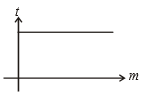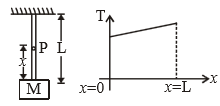# The length of a spring is ${l}_{1}$ $and$ ${l}_{2}$ when stretched with a force of 4 N and 5 N respectively. Its natural length is? 1. ${l}_{2}+{l}_{1}$ 2. $2\left({l}_{2}-{l}_{1}\right)$ 3. $5{l}_{1}-4{l}_{2}$ 4. $5{I}_{2}-4{l}_{1}$

Subtopic:  Spring Force |
To view explanation, please take trial in the course below.
NEET 2023 - Target Batch - Aryan Raj Singh
To view explanation, please take trial in the course below.
NEET 2023 - Target Batch - Aryan Raj Singh
Launched MCQ Practice Books

Prefer Books for Question Practice? Get NEETprep's Unique MCQ Books with Online Audio/Video/Text Solutions via Telegram Bot

A simple pendulum hangs from the roof of a train moving on horizontal rails. If the string is inclined towards the front of the train, then train is-

1. Moving with constant velocity

2. In accelerated motion

3. In retarded motion

4. At rest

Subtopic:  Application of Laws |
To view explanation, please take trial in the course below.
NEET 2023 - Target Batch - Aryan Raj Singh
Launched MCQ Practice Books

Prefer Books for Question Practice? Get NEETprep's Unique MCQ Books with Online Audio/Video/Text Solutions via Telegram Bot

The value of $$M$$ of the hanging block is in the figure, which will prevent the smaller block ($$m$$$$=$$$$1$$ kg) from slipping over the triangular block. All the surfaces are smooth and string and pulley are ideal. (Given: $$M'$$$$=4$$ kg and $$\theta$$ $$=37^\circ$$)1. $$12$$ kg
2. $$15$$ kg
3. $$10$$ kg
4. $$4$$ kg

Subtopic:  Pseudo Force |
To view explanation, please take trial in the course below.
NEET 2023 - Target Batch - Aryan Raj Singh
Launched MCQ Practice Books

Prefer Books for Question Practice? Get NEETprep's Unique MCQ Books with Online Audio/Video/Text Solutions via Telegram Bot

A lighter body and heavier body both are moving with same momentum and applied same retarding force. Their stoping distances are  respectively. Then the correct relation between -

1. ${s}_{1}>{s}_{2}$

2. ${s}_{1}<{s}_{2}$

3. ${s}_{1}={s}_{2}$

4. None of these

Subtopic:  Newton's Laws |
To view explanation, please take trial in the course below.
NEET 2023 - Target Batch - Aryan Raj Singh
To view explanation, please take trial in the course below.
NEET 2023 - Target Batch - Aryan Raj Singh
Launched MCQ Practice Books

Prefer Books for Question Practice? Get NEETprep's Unique MCQ Books with Online Audio/Video/Text Solutions via Telegram Bot

When a 5 kg plastic box is placed deep inside water, it accelerates up at a rate of g/6. How much sand should be put inside the box so that it may accelerate down at the rate of g/6?

1.  2kg

2.  3kg

3.  4kg

4.  5kg

Subtopic:  Application of Laws |
To view explanation, please take trial in the course below.
NEET 2023 - Target Batch - Aryan Raj Singh
To view explanation, please take trial in the course below.
NEET 2023 - Target Batch - Aryan Raj Singh
Launched MCQ Practice Books

Prefer Books for Question Practice? Get NEETprep's Unique MCQ Books with Online Audio/Video/Text Solutions via Telegram Bot

Let $\theta$ denote the angular displacement of a simple pendulum oscillating in a vertical plane. If the mass of bob is m, the tension in the string is mgcos$\theta$-

1.  always

2.  never

3.  at extreme

4.  at mean position

Subtopic:  Non Uniform Vertical Circular Motion |
To view explanation, please take trial in the course below.
NEET 2023 - Target Batch - Aryan Raj Singh
To view explanation, please take trial in the course below.
NEET 2023 - Target Batch - Aryan Raj Singh
Launched MCQ Practice Books

Prefer Books for Question Practice? Get NEETprep's Unique MCQ Books with Online Audio/Video/Text Solutions via Telegram Bot

The graph for stopping time (t) versus mass (m) is shown in the figure. Then which one of the pairs is given?1.  Momentum, force

2.  K.E., force

3.  Velocity, force

4.  None of these

Subtopic:  Application of Laws |
To view explanation, please take trial in the course below.
NEET 2023 - Target Batch - Aryan Raj Singh
To view explanation, please take trial in the course below.
NEET 2023 - Target Batch - Aryan Raj Singh
Launched MCQ Practice Books

Prefer Books for Question Practice? Get NEETprep's Unique MCQ Books with Online Audio/Video/Text Solutions via Telegram Bot

A metal ring of mass m and radius R is placed on smooth horizontal table and is set rotating about its own axis in such a way that each part of the ring moves with a speed v. Tension in the ring is:

1. $\frac{m{v}^{2}}{R}$

2. $\frac{m{v}^{2}}{2R}$

3. $\frac{m{v}^{2}}{2\mathrm{\pi }R}$

4. None of these

Subtopic:  Uniform Circular Motion |
To view explanation, please take trial in the course below.
NEET 2023 - Target Batch - Aryan Raj Singh
To view explanation, please take trial in the course below.
NEET 2023 - Target Batch - Aryan Raj Singh
Launched MCQ Practice Books

Prefer Books for Question Practice? Get NEETprep's Unique MCQ Books with Online Audio/Video/Text Solutions via Telegram Bot

Tension on the string at point P is T. The graph for tension (T) versus x is shown in the figure. Then the string is:1.  massless

2.  massfull

3.  tension on every point on the string is same when the string is having finite mass 4

4.  None of these

Subtopic:  Application of Laws |
To view explanation, please take trial in the course below.
NEET 2023 - Target Batch - Aryan Raj Singh
To view explanation, please take trial in the course below.
NEET 2023 - Target Batch - Aryan Raj Singh
Launched MCQ Practice Books

Prefer Books for Question Practice? Get NEETprep's Unique MCQ Books with Online Audio/Video/Text Solutions via Telegram Bot

While walking on ice one should take small steps to avoid slipping. This is because smaller steps ensure

1. Larger friction

2. Smaller friction

3. Larger normal force

4. Smaller normal force

Subtopic:  Application of Laws |
To view explanation, please take trial in the course below.
NEET 2023 - Target Batch - Aryan Raj Singh
To view explanation, please take trial in the course below.
NEET 2023 - Target Batch - Aryan Raj Singh